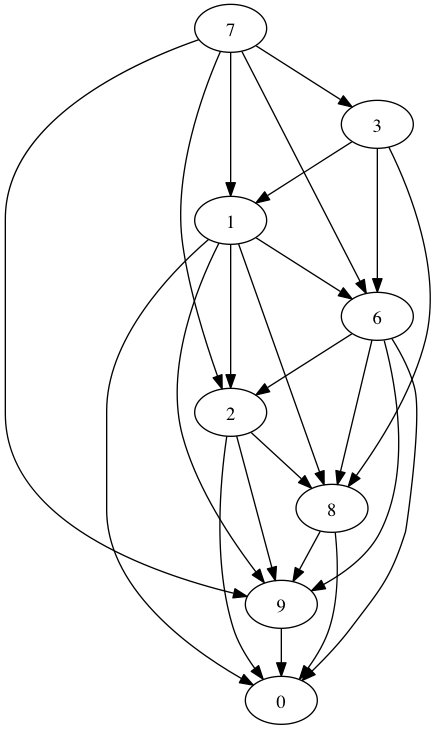# Fun Python Solution to Euler Problem 79

Nothing says Merry Christmas like Project Euler.

Here’s a nifty solution to Problem 79 that uses Python and Graphviz.

The problem is to identify a user’s password given a bunch of successful logins (taken from some kind of nefarious keylogger–those crafty devils). The Ã§atch is that each login is actually a subset of the actual password. Of course, they give you a sample of 50 successful logins.

So I decided to whip up some Python code to ingest each login and store a list of digit precedence. Then, the code prints out a Dot file which we turn into an image using the “dot” command. I ran this on Mac OS X, but it should work just fine on any Linux box (hint: install the “graphviz” package).

Here’s the Python code:

problem79.py:

```digit_precendence = dict()

for line in [line.strip() for line in open("./keylog.txt")]:
for index, char in enumerate(line):
digits = digit_precendence.setdefault(int(char), set())
if index < len(line)-1:

print "digraph problem79 {"
for (digit, subsequent_digits) in digit_precendence.iteritems():
for subsequent_digit in subsequent_digits:
print "   %d -> %d;" % (digit, subsequent_digit)
print "}"
```

Save that as problem79.py, download keylog.txt into the same folder, and run the program like this:

`python problem79.py | dot -Tpng > problem79.png`

Then view problem79.png, and voila! Graphviz just put the answer right in front of your nose: 73162890.